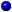HREF="s9node2.html">9.2 Programming in JAVA  Up: 9 LEARNING LABORATORY ENVIRONEMENT  Next: 9.4 Quick intermediate evaluation

9.3 VMarket parameters and preset in HTML

Slide : [ || VMARKET tree - names || JAVA tutorial ]Problem defining selectors.

The selectors appear on the top of VMARKET plot window and allow you to define the type of problem you want to solve. Careful, white spaces here do count!
• topic selects the type of the financial product. Choices include
"StckOption", "ZeroCpBond", "BondOption", "IRateSwap", "CreditModel", "RandomWalk", "Exercise".
• method selects the numerical method. Choices include
"FinDifferen", "FinElements", "Monte-Carlo", "Monte-Carlo*", "DistribFct", "DistribFct*".
• scheme selects the flavour of a given product and method. Choices include
"European", "European logn", "American", "American logn", "inBarrier", "outBarrier", "particles", "Binom tree", "Exercise 1.01".
• ic selects the type of initial or terminal condition. Choices include
"Put", "Call", "VSpread", "SuperShr", "Floorlet", "Caplet", "Gaussian".Editable run parameters.

The following list of parameters are first preset to default values, then modified according to the TAG parameters (below) and can be modified at run time by double-clicking the name appearing on the left of the applet:
• RunTime the run time [years], e.g. 0.25 for 3 months
• Drift the relative total drift of the underlying [1/year], e.g. 0.05 for 5%
• Volatility the relative volatility of the undelying [1/year], e.g. 0.5 for 50%
• LogNkappa exponent in the random walk, e.g. 1=log- / 0=normal
• SpotRate the present short term interest (spot) rate [1/year], e.g. 0.03 for 3%
• Dividend dividend yield [1/years], e.g. 0.04 for 4%
• StrikePrice the option exercise price at expiry [currency], e.g. 10 for EUR 10
• Barrier relative to underlying, e.g. -0.1 for a barrier 10% below the spot price
• MktPriceRsk market price of risk, e.g. -0.5 for risk averse market
• MeanRevTarg target of mean reversion rate, e.g. 0.05 for 5%
• MeanRevVelo speed of mean reversion process, e.g. 2 for 1/2 year
• Shape0 shape parameter or the yield curve amplitude
• Shape1 shape parameter or the yield curve slope
• Shape2 shape parameter or the yield curve convexity
• MeshLeft the lower end of the price range [currency]
• MeshLength the price range [currency]
• MeshPoint the number of mesh points
• Walkers the number of random walkers
• TimeStep the step [1/year], e.g. 0.00274 for one day, 0.01923 for one week
• TimeTheta the implicity parameter for time integration
• UserInteger the user defined integer value
• UserDouble the user defined double value
Only the parameters specified in the applet TAG are initially displayed; switch from Double-click below: to Show all parameters to get a complete listApplet TAG modifiers.

The VMARKET applet is included an HTML document with a specific header: the first couple of lines specify the path name of the executable, the position and the size of the window where the applet will appear. The TAG modifiers that follow defines the list of parameters that will be displayed and attributes default values when the applet is first initialized
<applet codebase="\$user_dir/applet/" code=\$applet
align=center width=780 height=420>
<param name=topic               value="Exercise">
<param name=scheme              value="Exercise 4.07">
<param name=ic                  value="Put">
<param name=method              value="FinDifferen">
<param name=RunTime             value= 0.5>
<param name=Drift               value= 0.>
<param name=Volatility          value= 0.4>
<param name=LogNkappa           value= 1.>
<param name=SpotRate            value= 0.1>
<param name=Dividend            value= 0.>
<param name=StrikePrice         value= 10.>
<param name=Barrier             value= 0.>
<param name=MktPriceRsk         value= 0.>
<param name=MeanRevTarg         value= 0.05>
<param name=MeanRevVelo         value= 0.>
<param name=Shape0              value= 1.>
<param name=Shape1              value= 0.001>
<param name=Shape2              value=-0.05>
<param name=MeshLeft            value= 0.>
<param name=MeshLength          value= 20.>
<param name=MeshPoints          value= 21>
<param name=Walkers             value= 300>
<param name=TimeStep            value= 0.00397>
<param name=TimeTheta           value= 0.7>